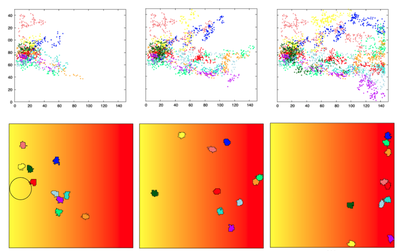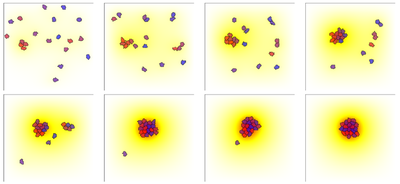# Chemotaxis

##### Persistent Identifier

Diffusion model with cells endowed with chemotactic ability

## Introduction

Previously developed diffusion models can be used to model chemotaxis by adding cells endowed with chemotactic ability. Here, chemotaxis of a group of cells towards a chemical diffusing into the domain with a high level at the right boundary is demonstrated, as well as in a second model, Keller-Segel cells being the sources of their own attractant.

## Description

Cells are endowed with the property of Chemotaxis with some rate $\chi$ towards a chemical $c$.

### Chemotaxis of Cells towards Domain Boundary

In SimpleChemotaxis_main.xml, the chemical level is initially high (BoundaryValue $= 5.0$) only along the right boundary of the domain. Diffusion and a DiffEqn for decay then result in the gradient from left to right that directs the cell motion.

### Keller-Segel Chemotaxis

In KellerSegelChemotaxis.xml, the cells are sources of their own attractant, and move by chemotaxis in response to that attractant. The secreted chemical then diffuses and decays in the domain.

## ResultsChemotaxis of cells towards a chemical attractant $c$ whose gradient is established by diffusion from the right boundary. Shown are the cells (bottom panels) at $t = 100, 200, 300$ time steps (left to right). The level of $c$ is indicated by red (high) to yellow (low) shades. Cells started at $t = 0$ in the small circle shown in the bottom left panel. The trajectories of the cells from $t = 0$ up to the given times are shown on the top panels. Produced with Morpheus file SimpleChemotaxis_main.xml.A simulation of the Keller-Segel chemotaxis, illustrating the aggregation of cells that produce a chemical and are attracted by it. A time sequence from left to right and top to bottom starting with time $t = 5$ in increments of $\Delta t = 15$. The level of the chemical attractant is shown in shades from white to yellow and red as its concentration increases. Cells are all identical, but colored differently for ease of tracking their motions. Produced by Morpheus file KellerSegelChemotaxis.xml.

## Model

Get this model via:

•  Download: SimpleChemotaxis_main.xml
• XML Preview

<?xml version='1.0' encoding='UTF-8'?>
<MorpheusModel version="4">
<Description>
<Details>Full title:		Chemotaxis
Authors:		L. Edelstein-Keshet
Contributors:	Y. Xiao
Date:		05.06.2022
Model ID:		https://identifiers.org/morpheus/M2010
File type:		Main model
Reference:		L. Edelstein-Keshet: Mathematical Models in Cell Biology
Comment:		Illustration of chemotaxis for a group of cells. A chemical c in a 2D domain, diffuses at rate D and decays at rate k. Periodic BCs in y direction, constant c on x boundaries, with high level at the right boundary. Heat map in 2D of the chemical to show its diffusion into the domain: Chemotaxis plug-in implemented in Morpheus. Cells have some attraction (with chemotaxis rate chi) towards the high chemical concentration. We also keep track of the cell trajectories over time to show the net drift towards the right.</Details>
<Title>SimpleChemotaxis</Title>
</Description>
<Space>
<Lattice class="square">
<Neighborhood>
<Order>1</Order>
</Neighborhood>
<Size symbol="size" value="150, 150, 0"/>
<BoundaryConditions>
<Condition type="constant" boundary="x"/>
<Condition type="constant" boundary="-x"/>
<Condition type="periodic" boundary="y"/>
</BoundaryConditions>
<NodeLength symbol="dx" value="0.1"/>
</Lattice>
<SpaceSymbol symbol="space"/>
</Space>
<Time>
<StartTime value="0"/>
<StopTime value="300"/>
<TimeSymbol symbol="time"/>
</Time>
<Global>
<Field symbol="c" name="attractant" value="0">
<Diffusion rate="0.5"/>
<BoundaryValue boundary="x" value="5.0"/>
</Field>
<System time-step="0.5" solver="Runge-Kutta [fixed, O(4)]">
<DiffEqn symbol-ref="c" name="attractant">
<Expression>-k*c</Expression>
</DiffEqn>
<Constant symbol="k" name="decay rate" value="0.001"/>
</System>
<Function symbol="x">
<Expression>dx*space.x</Expression>
</Function>
<Function symbol="y">
<Expression>dx*space.y</Expression>
</Function>
</Global>
<Analysis>
<!--    <Disabled>
<Logger time-step="5" name="chemical profile">
<Input>
<Symbol symbol-ref="c"/>
</Input>
<Output>
<TextOutput/>
</Output>
<Plots>
<Plot time-step="25" title="chemical profile">
<Style style="points"/>
<Terminal terminal="png"/>
<X-axis>
<Symbol symbol-ref="x"/>
</X-axis>
<Y-axis>
<Symbol symbol-ref="y"/>
</Y-axis>
<Color-bar>
<Symbol symbol-ref="c"/>
</Color-bar>
</Plot>
</Plots>
</Logger>
</Disabled>
-->
<Gnuplotter time-step="5">
<Plot title="chemotaxis of cells to chemical">
<Field symbol-ref="c">
<ColorMap>
<Color value="5" color="red"/>
<Color value="2" color="yellow"/>
</ColorMap>
</Field>
<Cells max="10" min="0" value="cell.id"/>
</Plot>
<Terminal name="png"/>
</Gnuplotter>
<ModelGraph format="svg" reduced="false" include-tags="#untagged"/>
<Logger time-step="1">
<Input>
<Symbol symbol-ref="cell.center.x"/>
<Symbol symbol-ref="cell.center.y"/>
</Input>
<Output>
<TextOutput/>
</Output>
<Plots>
<Plot time-step="50" title="Cell trajectories">
<Style point-size="0.5" style="points"/>
<Terminal terminal="png"/>
<X-axis minimum="0.0" maximum="150.0">
<Symbol symbol-ref="cell.center.x"/>
</X-axis>
<Y-axis minimum="0.0" maximum="150.0">
<Symbol symbol-ref="cell.center.y"/>
</Y-axis>
<Color-bar minimum="0.0" maximum="10.0">
<Symbol symbol-ref="cell.id"/>
</Color-bar>
</Plot>
</Plots>
</Logger>
</Analysis>
<CellTypes>
<CellType class="biological" name="neutrophil">
<Chemotaxis strength="chi" field="c&#xa;"/>
<Constant symbol="chi" name="chemotactic coefficient" value="1.0"/>
<VolumeConstraint target="50" strength="1"/>
<ConnectivityConstraint/>
</CellType>
<CellType class="medium" name="medium"/>
</CellTypes>
<CPM>
<Interaction>
<Contact type1="neutrophil" type2="neutrophil" value="8"/>
<Contact type1="neutrophil" type2="medium" value="4"/>
<!--    <Disabled>
<Contact type1="medium" type2="medium" value="0"/>
</Disabled>
-->
</Interaction>
<ShapeSurface scaling="norm">
<Neighborhood>
<Order>4</Order>
</Neighborhood>
</ShapeSurface>
<MonteCarloSampler stepper="edgelist">
<MCSDuration value="0.00375"/>
<MetropolisKinetics yield="0.1" temperature="2"/>
<Neighborhood>
<Order>2</Order>
</Neighborhood>
</MonteCarloSampler>
</CPM>
<CellPopulations>
<Population type="neutrophil" size="1">
<InitCircle number-of-cells="10" mode="random">
</InitCircle>
</Population>
</CellPopulations>
</MorpheusModel>